Question

# At a certain temperature and total pressure of 1.2 atm. the partial pressures of an equilibrium...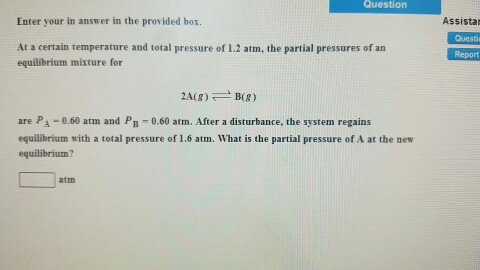At a certain temperature and total pressure of 1.2 atm. the partial pressures of an equilibrium mixture for 2A(g) B(g) are P_A - 0.60 atm and P_B - 0.60 aim. After a disturbance, the system regains equilibrium with a total pressure of 1.6 atm. What is the partial pressure of A at the equilibrium ? atm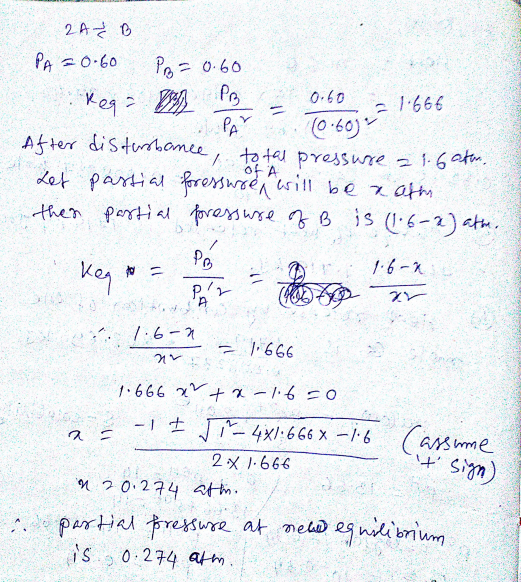#### Earn Coins

Coins can be redeemed for fabulous gifts.

Similar Homework Help Questions
• ### 7. (a) If the partial pressure of each component is 1 atm, and the temperature is...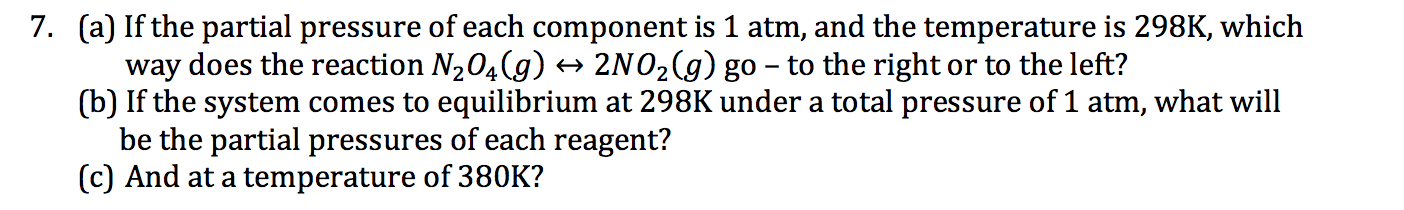7. (a) If the partial pressure of each component is 1 atm, and the temperature is 298K, which way does the reaction N204(g) + 2N02(g) go - to the right or to the left? (b) If the system comes to equilibrium at 298K under a total pressure of 1 atm, what will be the partial pressures of each reagent? (c) And at a temperature of 380K?

• ### A mixture of He, Ar, and Xe has a total pressure of 2.80 atm . The partial pressure of He is 0.300 atm , and the partial...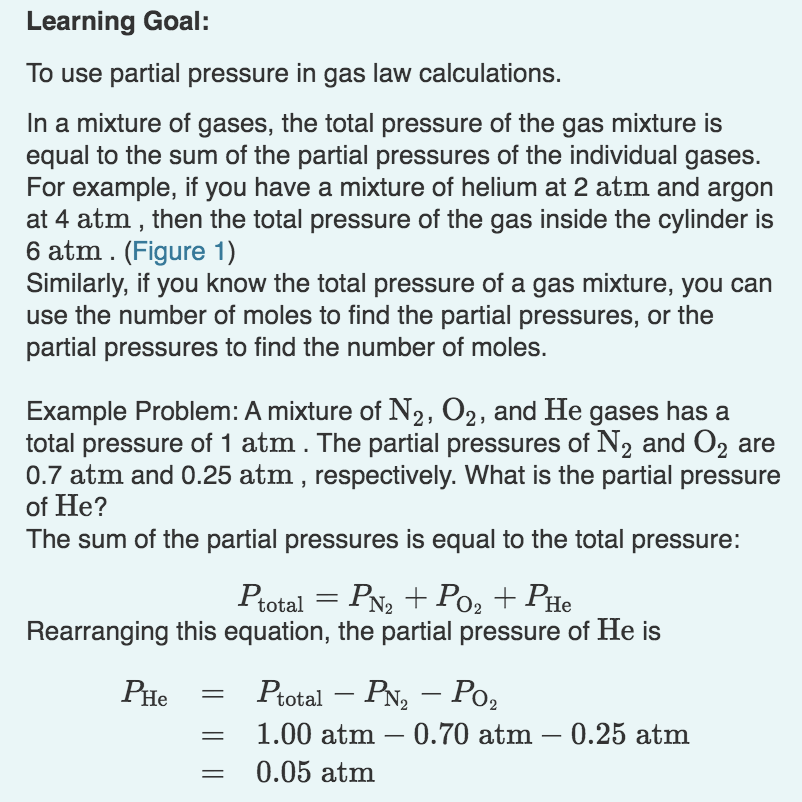A mixture of He, Ar, and Xe has a total pressure of 2.80 atm . The partial pressure of He is 0.300 atm , and the partial pressure of Ar is 0.300 atm . What is the partial pressure of Xe? A volume of 18.0 L contains a mixture of 0.250 mole N2 , 0.250 mole O2 , and an unknown quantity of He. The temperature of the mixture is 0 ∘C , and the total pressure is 1.00 atm...

• ### Calculate Kp if the total pressure of the system at equilibrium is 2.2 atm at that temperature

Consider the decomposition equilibrium of ammonium chloride at a certain temperature:NH4Cl(s) ? NH3(g) + HCl(g)Calculate Kp if the total pressure of the system at equilibrium is 2.2 atm at that temperature.A. 4.8B. 0.54C. 1.5D. 1.1E. 1.2

• ### 25. An equilibrium mixture of PCl5(g), PCl3(g), and Cl2(g) has partial pressures of 217.0 Torr, 13.2...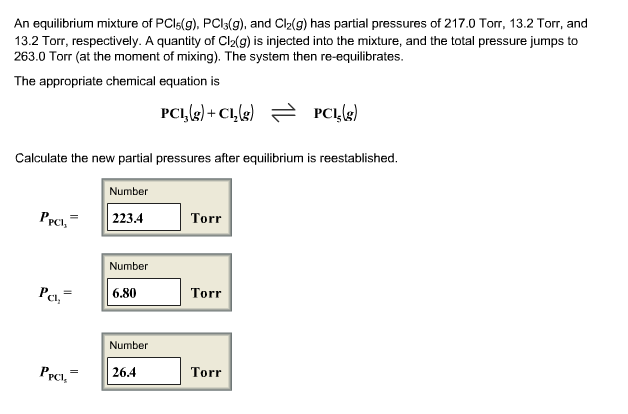25. An equilibrium mixture of PCl5(g), PCl3(g), and Cl2(g) has partial pressures of 217.0 Torr, 13.2 Torr, and 13.2 Torr, respectively. A quantity of Cl2(g) is injected into the mixture, and the total pressure jumps to 263.0 Torr (at the moment of mixing). The system then re-equilibrates. The appropriate chemical equation is Calculate the new partial pressures after equilibrium is reestablished. An equilibrium mixture of PCls(g), PCls(g), and Cl2(g) has partial pressures of 217.0 Torr, 13.2 Torr, and 13.2 Torr,...

• ### The equilibrium PCl5(g) ⇄ PCl3(g) + Cl2(g) is established at 250oC. At equilibrium the partial pressures...

The equilibrium PCl5(g) ⇄ PCl3(g) + Cl2(g) is established at 250oC. At equilibrium the partial pressures of the components are 0.020 Atm (PCl5), 1.28 Atm (PCl3), and 1.28 Atm (Cl2). If the partial pressure of Cl2 is suddenly increased to 2.15 Atm, what is the partial pressure of PCl5 after equilibrium has been reestablished?

• ### The elementary reaction proceeds at a certain temperature until the partial pressures of H2O, H2, and...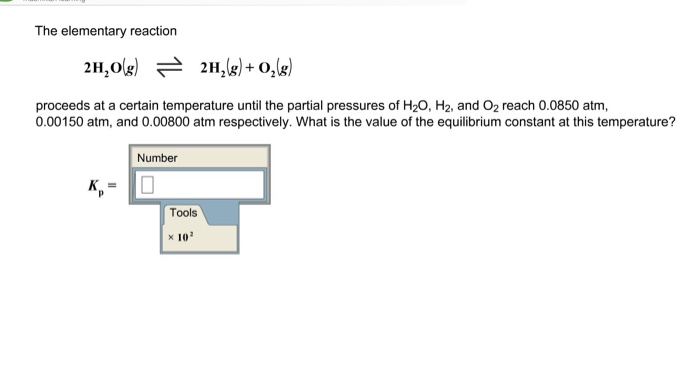The elementary reaction proceeds at a certain temperature until the partial pressures of H2O, H2, and O2 reach 0.0850 atm, 0.00150 atm, and 0.00800 atm respectively. What is the value of the equilibrium constant at this temperature? Number Tools x 10

• ### The elementary reaction 2H2O(g)−⇀↽−2H2(g)+O2(g) proceeds at a certain temperature until the partial pressures of H2O, H2,...

The elementary reaction 2H2O(g)−⇀↽−2H2(g)+O2(g) proceeds at a certain temperature until the partial pressures of H2O, H2, and O2 reach 0.0750 atm, 0.00850 atm, and 0.00650 atm, respectively. What is the value of the equilibrium constant at this temperature?

• ### The elementary reaction 2H20(g)<--->2H2(g)+O2(g) proceeds at a certain temperature until the partial pressures of H2O, H2,...

The elementary reaction 2H20(g)<--->2H2(g)+O2(g) proceeds at a certain temperature until the partial pressures of H2O, H2, and O2 reach 0.0700 atm, 0.00200 atm, and 0.00600 atm respectively. What is the value of the equilibrium constant at this temperature?

• ### An equilibrium mixture of PCI,(g), PCI, (8), and Cl, (g) has partial pressures of 217.0 Torr,...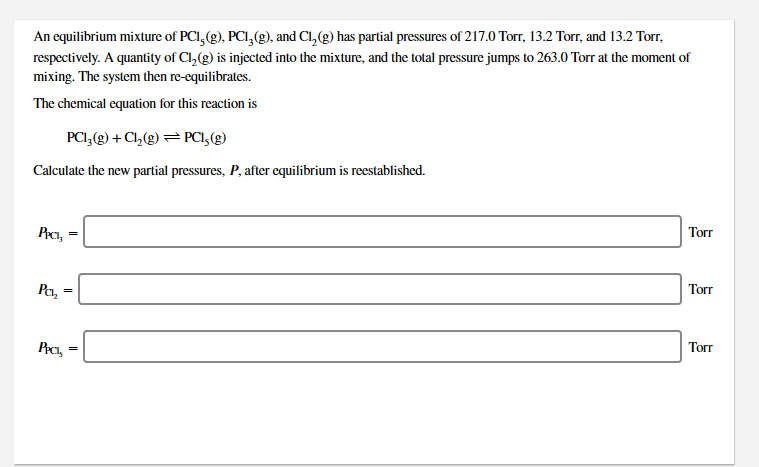An equilibrium mixture of PCI,(g), PCI, (8), and Cl, (g) has partial pressures of 217.0 Torr, 13.2 Torr, and 13.2 Torr, respectively. A quantity of Cl,g) is injected into the mixture, and the total pressure jumps to 263.0 Torr at the moment of mixing. The system then re-equilibrates. The chemical equation for this reaction is PCI,(g) +Cl (9) = PCI, (g) Calculate the new partial pressures, P, after equilibrium is reestablished. Pa- Bach, Torr

• ### Equilibrium partial pressures help

The following equilbrium partial pressures were measured at some temperatureNH3 N2 H20.290 atm 0.250 atm 0.340 atmDetermine the value of the equilibrium constant for the following reaction at the temperature.N2 + 3H2 2NH3K =In a different experiment at the same temperture, the equilibrium pressure of N2 was found to be 0.180atm and that of H2 to be 0.280 atm. What is the equilibriumpressure NH3 if K = 1.670 for the above reaction at the temperature of the equilibrium?P(NH3) = atmthanks